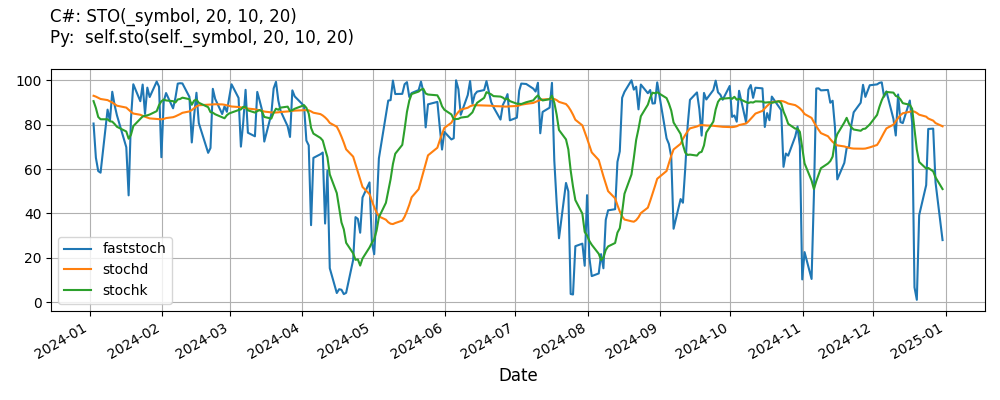# Supported Indicators

## Stochastic

### Introduction

This indicator computes the Slow Stochastics %K and %D. The Fast Stochastics %K is is computed by (Current Close Price - Lowest Price of given Period) / (Highest Price of given Period - Lowest Price of given Period) multiplied by 100. Once the Fast Stochastics %K is calculated the Slow Stochastic %K is calculated by the average/smoothed price of of the Fast %K with the given period. The Slow Stochastics %D is then derived from the Slow Stochastics %K with the given period.

To view the implementation of this indicator, see the LEAN GitHub repository.

### Using STO Indicator

To create an automatic indicators for Stochastic, call the STO helper method from the QCAlgorithm class. The STO method creates a Stochastic object, hooks it up for automatic updates, and returns it so you can used it in your algorithm. In most cases, you should call the helper method in the Initialize method.

public class StochasticAlgorithm : QCAlgorithm
{
private Symbol _symbol;
private Stochastic _sto;

public override void Initialize()
{
_sto = STO(_symbol, 20, 10, 20);
}

public override void OnData(Slice data)
{
{
// The current value of _sto is represented by itself (_sto)
// or _sto.Current.Value
Plot("Stochastic", "sto", _sto);
// Plot all properties of sto
Plot("Stochastic", "faststoch", _sto.FastStoch);
Plot("Stochastic", "stochk", _sto.StochK);
Plot("Stochastic", "stochd", _sto.StochD);
}
}
}
class StochasticAlgorithm(QCAlgorithm):
def Initialize(self) -> None:
self.sto = self.STO(self.symbol, 20, 10, 20)

def OnData(self, slice: Slice) -> None:
# The current value of self.sto is represented by self.sto.Current.Value
self.Plot("Stochastic", "sto", self.sto.Current.Value)
# Plot all attributes of self.sto
self.Plot("Stochastic", "faststoch", self.sto.FastStoch.Current.Value)
self.Plot("Stochastic", "stochk", self.sto.StochK.Current.Value)
self.Plot("Stochastic", "stochd", self.sto.StochD.Current.Value)


The following reference table describes the STO method:

### STO()1/2

            Stochastic QuantConnect.Algorithm.QCAlgorithm.STO (
Symbol                            symbol,
Int32                             period,
Int32                             kPeriod,
Int32                             dPeriod,
*Nullable<Resolution>       resolution,
*Func<IBaseData, TradeBar>  selector
)


Creates a new Stochastic indicator.

### STO()2/2

            Stochastic QuantConnect.Algorithm.QCAlgorithm.STO (
Symbol                            symbol,
Int32                             period,
*Nullable<Resolution>       resolution,
*Func<IBaseData, TradeBar>  selector
)


Overload short hand to create a new Stochastic indicator; defaulting to the 3 period for dStoch.

If you don't provide a resolution, it defaults to the security resolution. If you provide a resolution, it must be greater than or equal to the resolution of the security. For instance, if you subscribe to hourly data for a security, you should update its indicator with data that spans 1 hour or longer.

You can manually create a Stochastic indicator, so it doesn't automatically update. Manual indicators let you update their values with any data you choose.

Updating your indicator manually enables you to control when the indicator is updated and what data you use to update it. To manually update the indicator, call the Update method with a TradeBar, or QuoteBar. The indicator will only be ready after you prime it with enough data.

public class StochasticAlgorithm : QCAlgorithm
{
private Symbol _symbol;
private Stochastic _sto;

public override void Initialize()
{
_sto = new Stochastic(20, 10, 20);
}

public override void OnData(Slice data)
{
if (data.Bars.TryGeValue(_symbol, out var bar))
{
_sto.Update(bar);
}

{
// The current value of _sto is represented by itself (_sto)
// or _sto.Current.Value
Plot("Stochastic", "sto", _sto);
// Plot all properties of sto
Plot("Stochastic", "faststoch", _sto.FastStoch);
Plot("Stochastic", "stochk", _sto.StochK);
Plot("Stochastic", "stochd", _sto.StochD);
}
}
}
class StochasticAlgorithm(QCAlgorithm):
def Initialize(self) -> None:
self.sto = Stochastic(20, 10, 20)

def OnData(self, slice: Slice) -> None:
bar = slice.Bars.get(self.symbol)
if bar:
self.sto.Update(bar)

# The current value of self.sto is represented by self.sto.Current.Value
self.Plot("Stochastic", "sto", self.sto.Current.Value)
# Plot all attributes of self.sto
self.Plot("Stochastic", "faststoch", self.sto.FastStoch.Current.Value)
self.Plot("Stochastic", "stochk", self.sto.StochK.Current.Value)
self.Plot("Stochastic", "stochd", self.sto.StochD.Current.Value)


To register a manual indicator for automatic updates with the security data, call the RegisterIndicator method.

public class StochasticAlgorithm : QCAlgorithm
{
private Symbol _symbol;
private Stochastic _sto;

public override void Initialize()
{
_sto = new Stochastic(20, 10, 20);
RegisterIndicator(_symbol, _sto, Resolution.Daily);
}

public override void OnData(Slice data)
{
{
// The current value of _sto is represented by itself (_sto)
// or _sto.Current.Value
Plot("Stochastic", "sto", _sto);
// Plot all properties of sto
Plot("Stochastic", "faststoch", _sto.FastStoch);
Plot("Stochastic", "stochk", _sto.StochK);
Plot("Stochastic", "stochd", _sto.StochD);
}
}
}
class StochasticAlgorithm(QCAlgorithm):
def Initialize(self) -> None:
self.sto = Stochastic(20, 10, 20)
self.RegisterIndicator(self.symbol, self.sto, Resolution.Daily)

def OnData(self, slice: Slice) -> None:
# The current value of self.sto is represented by self.sto.Current.Value
self.Plot("Stochastic", "sto", self.sto.Current.Value)
# Plot all attributes of self.sto
self.Plot("Stochastic", "faststoch", self.sto.FastStoch.Current.Value)
self.Plot("Stochastic", "stochk", self.sto.StochK.Current.Value)
self.Plot("Stochastic", "stochd", self.sto.StochD.Current.Value)


The following reference table describes the Stochastic constructor:

### Stochastic()1/2

            Stochastic QuantConnect.Indicators.Stochastic (
string  name,
int     period,
int     kPeriod,
int     dPeriod
)


Creates a new Stochastics Indicator from the specified periods.

### Stochastic()2/2

            Stochastic QuantConnect.Indicators.Stochastic (
int  period,
int  kPeriod,
int  dPeriod
)


Creates a new Stochastic indicator from the specified inputs.

### Visualization

The following image shows plot values of selected properties of Stochastic using the plotly library.You can also see our Videos. You can also get in touch with us via Discord.